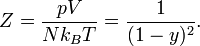# Scaled-particle theory$Z = \frac{ p V}{N k_B T} = \frac{ 1 }{(1-y)^2 }.$